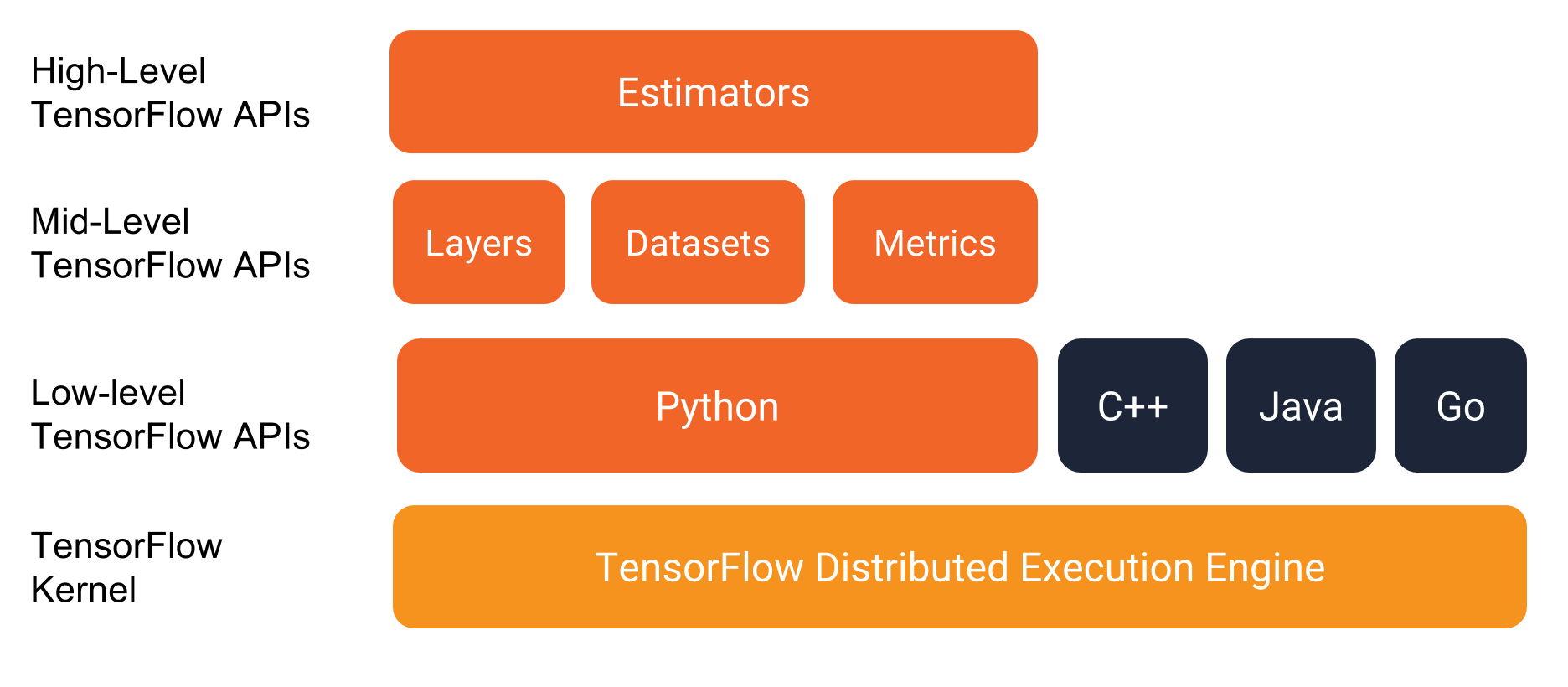# What's the difference between a Tensorflow Keras Model and Estimator?

Both Tensorflow Keras models and Tensorflow Estimators are able to train neural network models and use them to predict new data. They are both high-level APIs that sits on top of the low-level core TensorFlow API. So when should I use one over the other?

## Background

The Estimators API was added to Tensorflow in Release 1.1, and provides a high-level abstraction over lower-level Tensorflow core operations. It works with an Estimator instance, which is TensorFlow's high-level representation of a complete model.Keras is similar to the Estimators API in that it abstracts deep learning model components such as layers, activation functions and optimizers, to make it easier for developers. It is a model-level library, and does not handle low-level operations, which is the job of tensor manipulation libraries, or backends. Keras supports three backends - Tensorflow, Theano and CNTK.

Keras was not part of Tensorflow until Release 1.4.0 (2 Nov 2017). Now, when you use `tf.keras` (or talk about 'Tensorflow Keras'), you are simply using the Keras interface with the Tensorflow backend to build and train your model.So both the Estimator API and Keras API provides a high-level API over low-level core Tensorflow API, and you can use either to train your model. But in most cases, if you are working with Tensorflow, you'd want to use the Estimators API for the reasons listed below.

## Distribution

You can conduct distributed training across multiple servers with the Estimators API, but not with Keras API.

From the Tensorflow Keras Guide, it says that:

The Estimators API is used for training models for distributed environments.

And from the Tensorflow Estimators Guide, it says that:

You can run Estimator-based models on a local host or on a distributed multi-server environment without changing your model. Furthermore, you can run Estimator-based models on CPUs, GPUs, or TPUs without recoding your model.

Whilst Keras provides abstractions that makes building your models easier, you still have to write code to build your model. With Estimators, Tensorflow provides Pre-made Estimators, which are models which you can use straight away, simply by plugging in the hyperparameters.

Pre-made Estimators are similar to how you'd work with `scikit-learn`. For example, the `tf.estimator.LinearRegressor` from Tensorflow is similar to the `sklearn.linear_model.LinearRegression` from `scikit-learn`.

## Integration with Other Tensorflow Tools

Tensorflow provides a vistualzation tool called TensorBoard that helps you visualize your graph and statistics. By using an Estimator, you can easily save summaries to be visualized with Tensorboard.

## Converting Keras Model to Estimator

To migrate a Keras model to an Estimator, use the `tf.keras.estimator.model_to_estimator` method.

• PS: Keras does handle low level operations, it's just not very standard. Its backend (`import keras.backend as K`) contains lots of functions that wrap around the backend functions. They're meant to be used in custom layers, custom metrics, custom loss functions, etc. – Daniel Möller Jul 21 '18 at 12:08
• @DanielMöller thanks for your comment! Feel free to edit my answer if you'd like. – d4nyll Jul 21 '18 at 12:10
• While what you state is true, it ~seems~ that TF favors `tf.keras` given the amount of `colab` / documentation (of what little exists) is written with `tf.keras` over "canned" `tf.estimator.Estimator` or custom estimators – SumNeuron Nov 17 '18 at 23:02
• tf.estimator is built on top of Keras – prosti Jan 16 '19 at 10:00
• The Keras API is actually more supported than the Estimator API in terms of distributed training. You can read more here: tensorflow.org/guide/distributed_training. – hwaxxer Sep 30 '19 at 13:54

In my understanding, estimator is for training data on large scale and serving on production purpose, because cloud ML engine can only accept estimator.

The description below from one of tensorflow doc mentioned this:

" The Estimators API is used for training models for distributed environments. This targets industry use cases such as distributed training on large datasets that can export a model for production. "